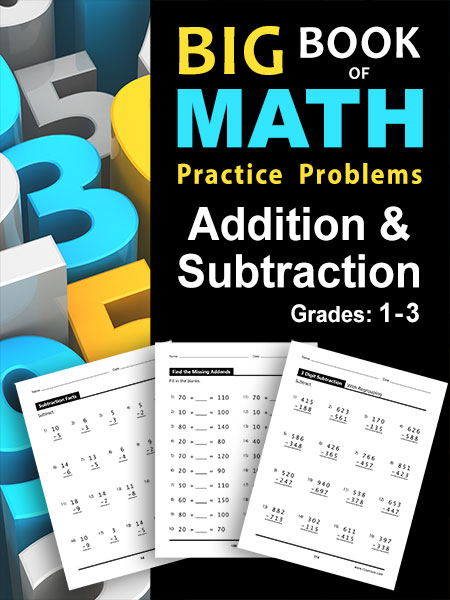ClayMaze.com

Big Book of Math Practice Problems, a great STEM Learning Resource
Over 4,000 Addition and Subtraction practice problems.

Big Book of Math Practice Problems Addition and SubtractionBig Book of Math Practice Problems Addition and Subtraction: Single Digit Facts / Drills, Double Digits, Triple Digits, Arithmetic With & Without Regrouping